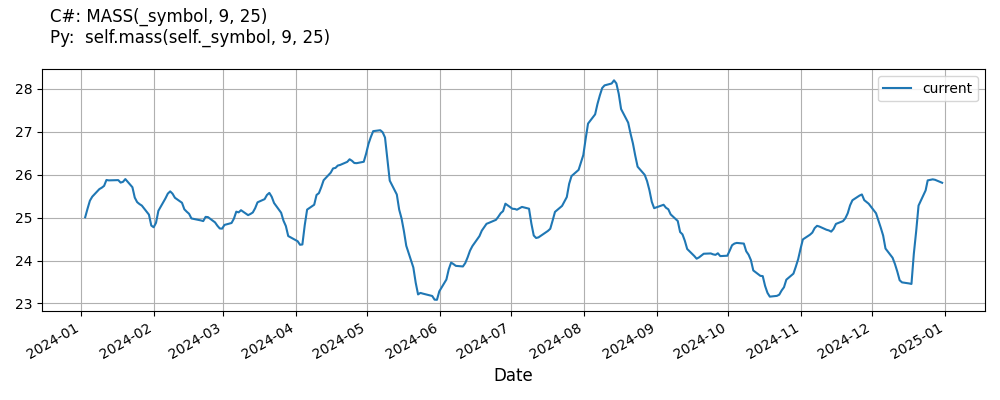# Supported Indicators

## Mass Index

### Introduction

The Mass Index uses the high-low range to identify trend reversals based on range expansions. In this sense, the Mass Index is a volatility indicator that does not have a directional bias. Instead, the Mass Index identifies range bulges that can foreshadow a reversal of the current trend. Developed by Donald Dorsey.

To view the implementation of this indicator, see the LEAN GitHub repository.

### Using MASS Indicator

To create an automatic indicators for MassIndex, call the MASS helper method from the QCAlgorithm class. The MASS method creates a MassIndex object, hooks it up for automatic updates, and returns it so you can used it in your algorithm. In most cases, you should call the helper method in the Initialize method.

public class MassIndexAlgorithm : QCAlgorithm
{
private Symbol _symbol;
private MassIndex _mass;

public override void Initialize()
{
_mass = MASS(_symbol, 9, 25);
}

public override void OnData(Slice data)
{
{
// The current value of _mass is represented by itself (_mass)
// or _mass.Current.Value
Plot("MassIndex", "mass", _mass);

}
}
}
class MassIndexAlgorithm(QCAlgorithm):
def Initialize(self) -> None:
self.mass = self.MASS(self.symbol, 9, 25)

def OnData(self, slice: Slice) -> None:
# The current value of self.mass is represented by self.mass.Current.Value
self.Plot("MassIndex", "mass", self.mass.Current.Value)



The following reference table describes the MASS method:

### MASS()1/1

            MassIndex QuantConnect.Algorithm.QCAlgorithm.MASS (
Symbol                            symbol,
*Int32                            emaPeriod,
*Int32                            sumPeriod,
*Nullable<Resolution>       resolution,
*Func<IBaseData, TradeBar>  selector
)


Creates a new Mass Index indicator. The indicator will be automatically updated on the given resolution.

If you don't provide a resolution, it defaults to the security resolution. If you provide a resolution, it must be greater than or equal to the resolution of the security. For instance, if you subscribe to hourly data for a security, you should update its indicator with data that spans 1 hour or longer.

You can manually create a MassIndex indicator, so it doesn't automatically update. Manual indicators let you update their values with any data you choose.

Updating your indicator manually enables you to control when the indicator is updated and what data you use to update it. To manually update the indicator, call the Update method with a TradeBar. The indicator will only be ready after you prime it with enough data.

public class MassIndexAlgorithm : QCAlgorithm
{
private Symbol _symbol;
private MassIndex _mass;

public override void Initialize()
{
_mass = new MassIndex(9, 25);
}

public override void OnData(Slice data)
{
if (data.Bars.TryGeValue(_symbol, out var bar))
{
_mass.Update(bar);
}

{
// The current value of _mass is represented by itself (_mass)
// or _mass.Current.Value
Plot("MassIndex", "mass", _mass);

}
}
}
class MassIndexAlgorithm(QCAlgorithm):
def Initialize(self) -> None:
self.mass = MassIndex(9, 25)

def OnData(self, slice: Slice) -> None:
bar = slice.Bars.get(self.symbol)
if bar:
self.mass.Update(bar)

# The current value of self.mass is represented by self.mass.Current.Value
self.Plot("MassIndex", "mass", self.mass.Current.Value)



To register a manual indicator for automatic updates with the security data, call the RegisterIndicator method.

public class MassIndexAlgorithm : QCAlgorithm
{
private Symbol _symbol;
private MassIndex _mass;

public override void Initialize()
{
_mass = new MassIndex(9, 25);
RegisterIndicator(_symbol, _mass, Resolution.Daily);
}

public override void OnData(Slice data)
{
{
// The current value of _mass is represented by itself (_mass)
// or _mass.Current.Value
Plot("MassIndex", "mass", _mass);

}
}
}
class MassIndexAlgorithm(QCAlgorithm):
def Initialize(self) -> None:
self.mass = MassIndex(9, 25)
self.RegisterIndicator(self.symbol, self.mass, Resolution.Daily)

def OnData(self, slice: Slice) -> None:
# The current value of self.mass is represented by self.mass.Current.Value
self.Plot("MassIndex", "mass", self.mass.Current.Value)



The following reference table describes the MassIndex constructor:

### MassIndex()1/2

            MassIndex QuantConnect.Indicators.MassIndex (
string  name,
int     emaPeriod,
int     sumPeriod
)


Initializes a new instance of the MassIndex class.

### MassIndex()2/2

            MassIndex QuantConnect.Indicators.MassIndex (
*int  emaPeriod,
*int  sumPeriod
)


Initializes a new instance of the MassIndex class.

### Visualization

The following image shows plot values of selected properties of MassIndex using the plotly library.You can also see our Videos. You can also get in touch with us via Discord.